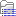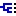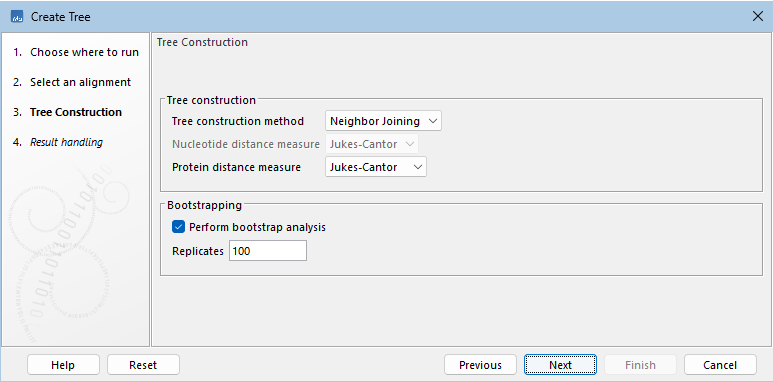# Create tree

The Create tree tool can be used to generate a distance-based phylogenetic tree with multiple alignments as input:

Toolbox | Classical Sequence Analysis () | Alignments and Trees ()| Create Tree ()

This will open the dialog displayed in figure 23.3:Figure 23.3: Creating a tree.

If an alignment was selected before choosing the Toolbox action, this alignment is now listed in the Selected Elements window of the dialog. Use the arrows to add or remove elements from the Navigation Area. Click Next to adjust parameters.Figure 23.4: Adjusting parameters for distance-based methods.

Figure 23.4 shows the parameters that can be set for this distance-based tree creation:

• Tree construction (see Distance-based methods)
• Tree construction method
• The UPGMA method. Assumes constant rate of evolution.
• The Neighbor Joining method. Well suited for trees with varying rates of evolution.
• Nucleotide distance measure
• Jukes-Cantor. Assumes equal base frequencies and equal substitution rates.
• Kimura 80. Assumes equal base frequencies but distinguishes between transitions and transversions.
• Protein distance measure
• Jukes-Cantor. Assumes equal amino acid frequency and equal substitution rates.
• Kimura protein. Assumes equal amino acid frequency and equal substitution rates. Includes a small correction term in the distance formula that is intended to give better distance estimates than Jukes-Cantor.

• Bootstrapping.
• Perform bootstrap analysis. To evaluate the reliability of the inferred trees, CLC Genomics Workbench allows the option of doing a bootstrap analysis (see Bootstrap tests). A bootstrap value will be attached to each node, and this value is a measure of the confidence in the subtree rooted at the node. The number of replicates used in the bootstrap analysis can be adjusted in the wizard. The default value is 100 replicates which is usually enough to distinguish between reliable and unreliable nodes in the tree. The bootstrap value assigned to each inner node in the output tree is the percentage (0-100) of replicates which contained the same subtree as the one rooted at the inner node.

For a more detailed explanation, see Bioinformatics explained.